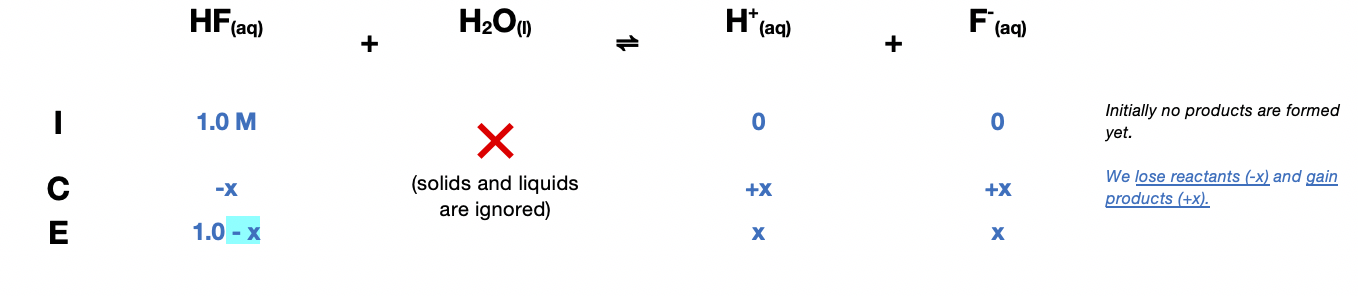# Problem: What mass of Ca(NO3)2 must be added to 1.0 L of a 1.0-M HF solution to begin precipitation of CaF2(s)? For CaF2, Ksp = 4.0 X 10 -11 and Ka for HF = 7.2 X 10 -4. Assume no volume change on addition of Ca(NO3)2(s).

###### FREE Expert Solution

Determine F- concentration first by constructing an ICE Chart.$\overline{){{\mathbf{K}}}_{{\mathbf{a}}}{\mathbf{=}}\frac{\mathbf{products}}{\mathbf{reactants}}{\mathbf{=}}\frac{\left[{H}^{+}\right]\left[{F}^{-}\right]}{\left[\mathrm{HF}\right]}}$

${\mathbf{7}}{\mathbf{.}}{\mathbf{2}}{\mathbf{×}}{{\mathbf{10}}}^{\mathbf{-}\mathbf{4}}{\mathbf{=}}\frac{\left(x\right)\left(x\right)}{\left(1.0-x\right)}$

${\mathbf{7}}{\mathbf{.}}{\mathbf{2}}{\mathbf{×}}{{\mathbf{10}}}^{\mathbf{-}\mathbf{4}}{\mathbf{=}}\frac{{x}^{2}}{\left(1.0-\overline{)x}\right)}\phantom{\rule{0ex}{0ex}}\phantom{\rule{0ex}{0ex}}{{\mathbf{x}}}^{{\mathbf{2}}}{\mathbf{=}}{\mathbf{7}}{\mathbf{.}}{\mathbf{2}}{\mathbf{×}}{{\mathbf{10}}}^{\mathbf{-}\mathbf{4}}\phantom{\rule{0ex}{0ex}}\phantom{\rule{0ex}{0ex}}\sqrt{{\mathbf{x}}^{\mathbf{2}}}{\mathbf{=}}\sqrt{\mathbf{7}\mathbf{.}\mathbf{2}\mathbf{×}{\mathbf{10}}^{\mathbf{-}\mathbf{4}}}$

x = [F-] = 0.0268 M

Calculate Ca2+:

90% (397 ratings)###### Problem Details

What mass of Ca(NO3)2 must be added to 1.0 L of a 1.0-M HF solution to begin precipitation of CaF2(s)? For CaF2, Ksp = 4.0 X 10 -11 and Ka for HF = 7.2 X 10 -4. Assume no volume change on addition of Ca(NO3)2(s).